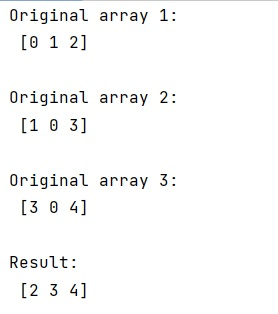# How to find element-wise maximum values from a NumPy array?

Learn, how to apply a function on NumPy arrays for finding maximum values that too element-wise?
Submitted by Pranit Sharma, on March 09, 2023

## NumPy Array: Finding element-wise maximum values

Suppose that we are given multiple NumPy arrays and we need to apply a max function on these arrays together in such a way that it checks each element and returns an array of the max element of each array.

To find element-wise maximum values from a NumPy array, we can use numpy.vstack() which will stack all the arrays vertically and then we will apply max() function with (axis = 0), since the arrays are stacked vertically. We need to extract max values from each column.

Let us understand with the help of an example,

## Python code to find element-wise maximum values from a NumPy array

```# Import numpy
import numpy as np

# Creating numpy arrays
A = np.array([0,1,2])
B = np.array([1,0,3])
C = np.array([3,0,4])

# Display Original arrays
print("Original array 1:\n",A,"\n")
print("Original array 2:\n",B,"\n")
print("Original array 3:\n",C,"\n")

# Stacking all the arrays vertically
res = np.vstack([A,B,C])

# Finding max value
max = res.max(axis=1)

# Display result
print("Result:\n",max)
```

### OutputLanguages: » C » C++ » C++ STL » Java » Data Structure » C#.Net » Android » Kotlin » SQL
Web Technologies: » PHP » Python » JavaScript » CSS » Ajax » Node.js » Web programming/HTML
Solved programs: » C » C++ » DS » Java » C#
Aptitude que. & ans.: » C » C++ » Java » DBMS
Interview que. & ans.: » C » Embedded C » Java » SEO » HR
CS Subjects: » CS Basics » O.S. » Networks » DBMS » Embedded Systems » Cloud Computing
» Machine learning » CS Organizations » Linux » DOS
More: » Articles » Puzzles » News/Updates